Next: Bibliography

Xian-Ming Gu
Nested Krylov subspace methods based on the Hessenberg procedure for shifted linear systems

Institute of Mathematics and Computing Science
University of Groningen
Nijenborgh 9
P O Box 407
9700 AK Groningen
The Netherlands
guxianming@live.cn
T.-Z. Huang
B. Carpentieri

In many computational applications it is required to solve simultaneously shifted linear systems of the form(1)

whereis a non-Hermitian matrix,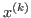is the unknown vector and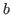is the right-hand side, both of them are complex vectors of length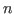. For instance, shifted linear systems arise in the solution of PageRank problems and the rational approximation of the action of a matrix function to a vector, i.e.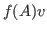, whereis an arbitrary vector with length.

In principle, a sequence of shifted linear systems (1) can be solved simultaneously at the cost of a single solve using shifted Krylov subspace methods (abbreviated as KSMs). These methods employ the property that Krylov subspaces are invariant under arbitrary diagonal shifts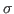to the matrix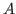, i.e.,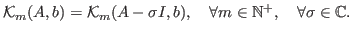(2)

As we know, the restarted GMRES method is a widely popular choice for solving (1), refer to . The computed GMRES shifted residuals are not collinear in general after the first restart so that it loses the computational efficiency mentioned above. Consequently, certain enforcement has to be made to guarantee the computed GMRES shifted residuals collinear to each other in order to maintain the computational efficiency. Note that in this case, only the seed system satisfies the minimum residual property, the solution of the other shifted systems is not equivalent to GMRES applied to those systems, see  for details. Moreover, another attractive approach is to use the restarted FOM method for solving the whole sequence of shifted linear systems simultaneously, for all residuals are naturally collinear [1,2]. As a result, the computational efficiency can be maintained because the orthonormal basis and the Hessenberg matrix are required to be calculated only once each time.

However, both the restarted GMRES method and the restarted FOM method for (1) are inherently derived by the Arnoldi procedure, which tends to be expensive when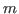becomes large in aspects of memory and algorithmic cost. To alleviate these difficulties, our recent work  proposed a new iterative strategy with collinear residuals for solving sequence of shifted linear systems based on the Hessenberg process, which is similar but cheaper compared to the Arnoldi procedure. Our theoretical and numerical analyses indicate that iterative schemes referred to as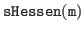may outperform the conventional shifted FOM method in aspects of iteration counts and CPU time. From the algorithmic property, it turns out that preserving orthonormality in the construction of the Krylov basis is sometimes not necessary for an efficient iterative solution of (1). This observation motivates us to extend the restarted Changing Minimal Residual method based on the Hessenberg process (CMRH) , which is similar to the restarted shifted GMRES method but much cheaper, for solving (1).

In practice, preconditioning shifted systems (1) is necessary to accelerate the convergence of shifted KSMs and this remains an open question, because standard preconditioning techniques may destroy the shift-invariance property (2). In this work, we construct an inner-outer scheme based on nested KSMs, where the inner solver is a multi-shift KSM (i.e.,) that acts as a preconditioner for an outer flexible CMRH (FCMRH) method. Our numerical experiments show that the new nested iterative scheme based on the Hessenberg procedure is very competitive, and sometimes superior, compared to the early established combination (FOM-FGMRES) in  in terms of CPU time. In summary, in our presentation first we discuss the derivations of both themethod  and the restarted shifted CMRH method. Then, we present their flexible variant combined with nested inner-outer iterative schemes, namely multi-shift Hessen-FCMRH. Meanwhile, extensive numerical experiments involving PageRank problems and numerical solutions of space(-time) fractional differential equations are reported to illustrate the effectiveness of the restarted shifted CMRH method and the Hessen-FCMRH method, respectively.Next: Bibliography
root 2016-02-22